# MOD Excel FunctionArticle byExcelMojo Team## What Is MOD Function In Excel?

The MOD function in Excel is an inbuilt Math & Trig function. It returns the remainder after dividing the given number by the specified divisor. And the resulting value carries the same sign as the divisor. Users can use the MOD Excel function to check if a cell contains an even or odd number and determine every nth row or column cell value required for calculations.

For example, the below table contains a list of numbers and the corresponding divisors to divide them with to get the required remainders.

And suppose the requirement is to determine and display the remainder values in column C. Then, considering the MOD Excel function definition, we can apply the MOD() in the target cells to get the required data.

The MOD Excel function return value in each target cell is the remainder resulting from dividing the given number by the specified divisor.

For example, the MOD Excel function in cell C2 accepts 500 and 100 as the arguments. Then, the number 500 gets divided by 100, and the remainder resulting from the division is 0. And thus, the MOD Excel function return value in cell C2 is 0.

###### Key Takeaways
• The MOD Excel function performs the modulo operation and determines the remainder after dividing the specified number with the given divisor.
• Users can use the MOD() to identify every nth row or column cell value for performing the required calculations with them. The function is also useful for highlighting odd and even numbers or multiples of a specified number.
• Remember, the MOD() takes two mandatory arguments, number, and divisor, as input.
• Using the MOD function with Excel functions such as IF, SUMPRODUCT, COLUMN, and ROW and the conditional formatting feature can yield fruitful results.

### MOD() Excel Formula

The MOD Excel function syntax is:

where,

• number: The value for which we require to determine the remainder.
• divisor: The value by which we need to divide the given number.

Both the MOD Excel function arguments are mandatory.

On the other hand, below are the critical aspects of the function we must know to avoid the possibility of the MOD Excel function not working.

• If the supplied arguments are not numbers, the MOD() will return the #VALUE! error.
• If the divisor is 0, the MOD() return value will be the #DIV/0! error.

### How To Use MOD Excel Function?

The steps to use the MOD Excel function are as follows:

1. First, confirm if the source data contains the numbers required to supply to the MOD() as arguments.
2. Next, select the target cell where we need to display the result and enter the MOD Excel function.
3. Finally, press Enter to view the remainder value.

The following example explains the above steps to ensure we avoid the condition of the MOD Excel function not working.

The below table lists a set of numbers and divisors.

And suppose we require to divide each number with the respective divisor and display the remainder in column C. Then, here is how we can apply the MOD() in the target cells and achieve the required outcome.

1. First, select the target cell C2, enter the MOD(), and then, press Enter.

=MOD(A2,B2)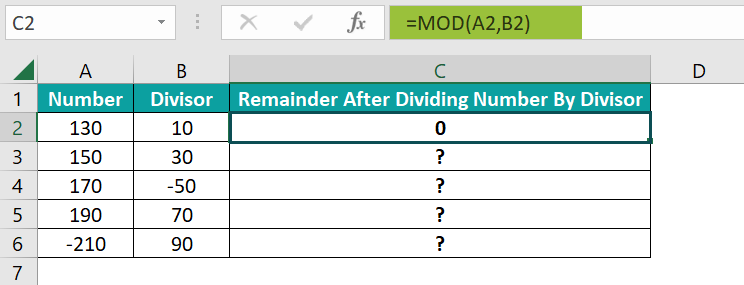Remember, directly entering the argument values will also give us the same result.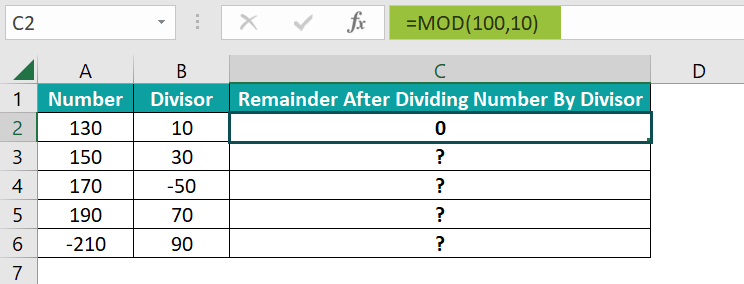Alternatively, we can select the target cell and click FormulasMath & TrigMOD to open the Function Arguments window. And we can enter the MOD() arguments from the Function Arguments window to apply the function in the target cell.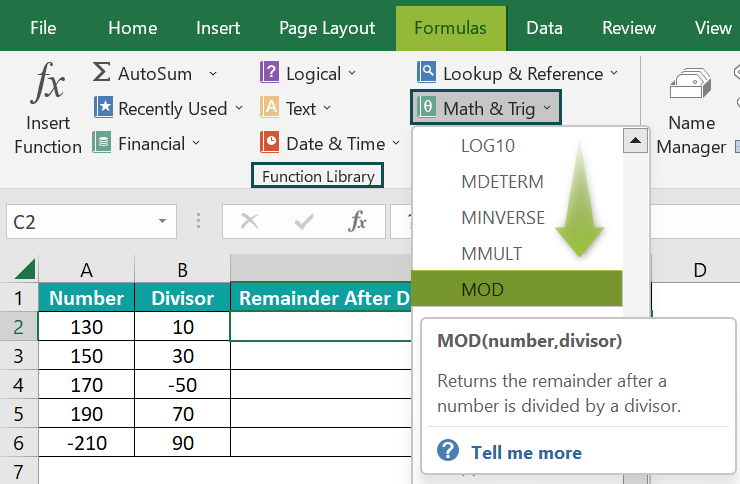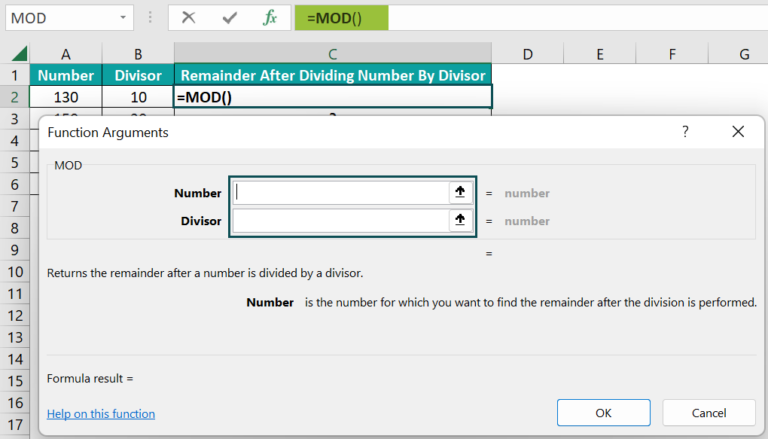And once we fill in the argument fields in the Function Arguments window, as shown below, press OK. Then, the MOD() will get executed in cell C2.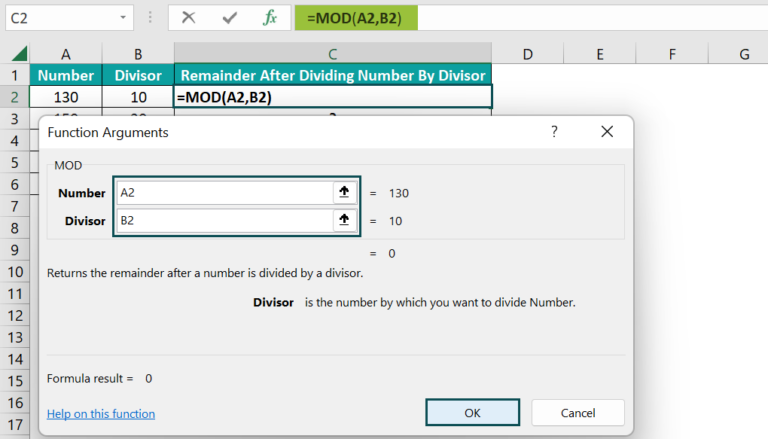We can also enter the number values directly in the argument fields instead of the cell references to get the required result.

2. Next, using the fill handle, apply the formula in cell range C3:C6.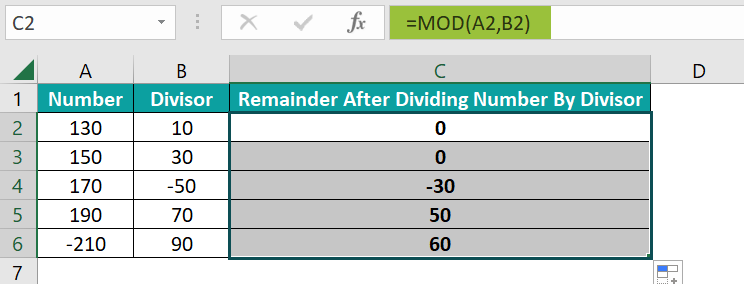### Examples of MOD Excel Function

This section shows examples to help you understand the MOD Excel function and use the function effectively.

#### Example #1

This example explains the scenario of the MOD Excel function multiple conditions.

The table below contains a list of fruits and their order details.

Suppose we must check the February month order data for positive and negative order quantities, date-wise, and display the output in column D.

Then, we can apply the MOD()with the IF excel function in the target cells to achieve the required data.

• Step 1: To begin with, select the target cell D2, enter the below formula, and press Enter.

=IF(MONTH(B2)=2,IF(MOD(C2,2)=0,”Even Order”,”Odd Order”),””)

The above formula involves the MOD Excel function multiple conditions.

• Step 2: Next, copy the above formula in cell range D3:D8 using the fill handle.

Now, let us consider the cell D8 formula to understand how it works.

First, the outer IF() checks if the date in cell B8 lies in February. As the condition holds, the inner IF() gets executed. So, the MOD() returns the remainder obtained from dividing the quantity specified in cell C8, 108, by 2, which is 0. And the IF() condition checks if the MOD() output equals 0.

Finally, as the condition holds, the IF() returns Even Order as the order check status in the specific target cell.

#### Example #2

We can use the MOD Excel function to determine every nth row or column value to perform calculations on them.

In this example, we shall add every nth-column value.

The first table contains the first and last six months’ sales data for different regional offices of a company.

Columns H and O show the sum of Jan-Jun and Jul-Dec sales figures for each regional office.

Suppose the requirement is to find the total sales at each regional office by adding the corresponding columns H and O values and display the result in the second table. Then, applying the MOD with the SUMPRODUCT excel function and COLUMN() in the target cells will help us get the required data.

• Step 1: First, select the target cell B10, enter the below formula, and then, press Enter.

=SUMPRODUCT((MOD(COLUMN(\$B2:\$O2)-COLUMN(\$B2)+1,7)=0)*(\$B2:\$O2))

Let us consider the cell B13 formula to understand how the formula works.

The first COLUMN excel formula returns the array of specified column range, {2,3,4,5,6,7,8,9,10,11,12,13,14,15}. And the second COLUMN() output is 2.

Here, the value 2 gets subtracted from each array value to return the difference between the two COLUMN() outputs, {0,1,2,3,4,5,6,7,8,9,10,11,12,13}. Next, adding the value 1 to the above array values results in {1,2,3,4,5,6,7,8,9,10,11,12,13,14}.

And then, the MOD() displays the remainders when each array value gets divided by 7, {1,2,3,4,5,6,0,1,2,3,4,5,6,0}.

Next, each resulting array value gets compared to 0, and we get an array of TRUE and FALSE values, with TRUE indicating the specific array value is 0 and FALSE otherwise.

The expression \$B5:\$O5 displays all the sales values in cells B5 to O5. And when the array values get multiplied by the cell range B5:O5 values, the output is {0,0,0,0,0,0,7970,0,0,0,0,0,0,9300}.

Finally, the SUMPRODUCT() adds the array values to return the required RG4 regional office total sales as \$17,270.

#### Example #3

This example shows how to use the MOD Excel function with the conditional formatting feature.

The below table contains a list of numbers.

Now, consider the requirement to highlight the multiples of the number specified in cell C2, 21, in column A.

Then, we can use the MOD Excel function in the conditional formatting criterion and highlight the required values in column A.

• Step 1: To begin with, select the cell range A2:A11 and then, click HomeConditional FormattingNew Rule to open the New Formatting Rule window.
• Step 2: Pick the last Rule Type. Next, enter the required criterion containing the MOD() in the provided field, and then, click Format to open the Format Cells window.
• Step 3: Next, go to the Fill tab, pick the color we wish to use for highlighting the cells where the conditional formatting criterion holds, and then, click OK.
• Step 4: Then, click OK in the New Formatting Rule window to view the highlighted cells.

The MOD() used in the conditional formatting criterion returns the remainder values resulting from dividing each column A number by 21. And the formatting condition highlights those cells in column A, for which the MOD() output is 0.

### Important Things To Note

• The MOD Excel function output will maintain the same sign as the divisor.
• Suppose we do not provide the two MOD() arguments as numbers. Then, the function will throw the #VALUE! error.
• When the supplied divisor argument value is 0, the MOD function output will be the #DIV/0! error.

1. Where is the MOD function in Excel?

The MOD function in Excel is in the Formulas tab. Click FormulasMath & TrigMOD to apply it in the required target cell.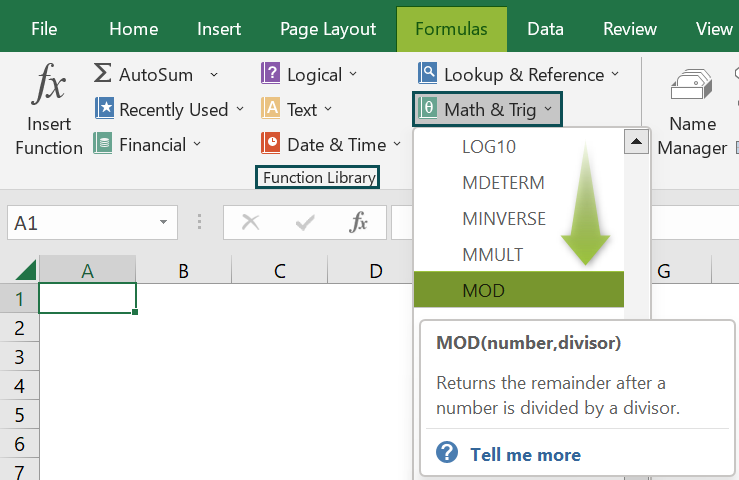2. What is the purpose of the MOD function in Excel?

The purpose of the MOD function in Excel is that as it gives the remainder from dividing the given number with the specified divisor, it helps identify every nth cell value. We can also use the function to determine odd and even numbers from the given number list.

3. How to use MOD() to count the number of cells containing even and odd numbers?

We can use MOD() to count the number of cells containing even and odd numbers by applying it along with the SUMPRODUCT function.

Let us see the steps with an example.

Consider the following table containing a list of even and odd numbers.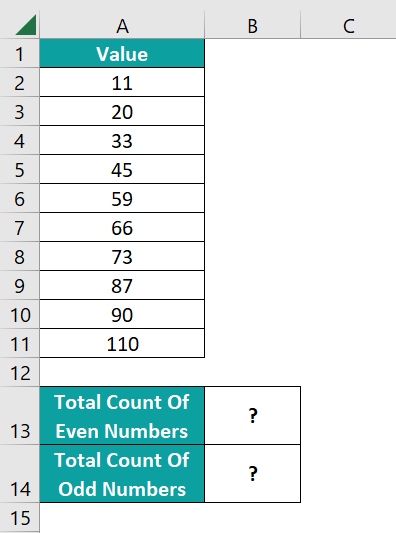Suppose the requirement is to determine the total number of cells containing even and odd numbers and display the results in the cell range B13:B14. Then, here is how we can use the MOD() within the SUMPRODUCT() in the target cells to get the required output.

• Step 1: Select the target cell B13, enter the below formula, and press Enter.
=SUMPRODUCT((MOD(A2:A11,2)=0)*1)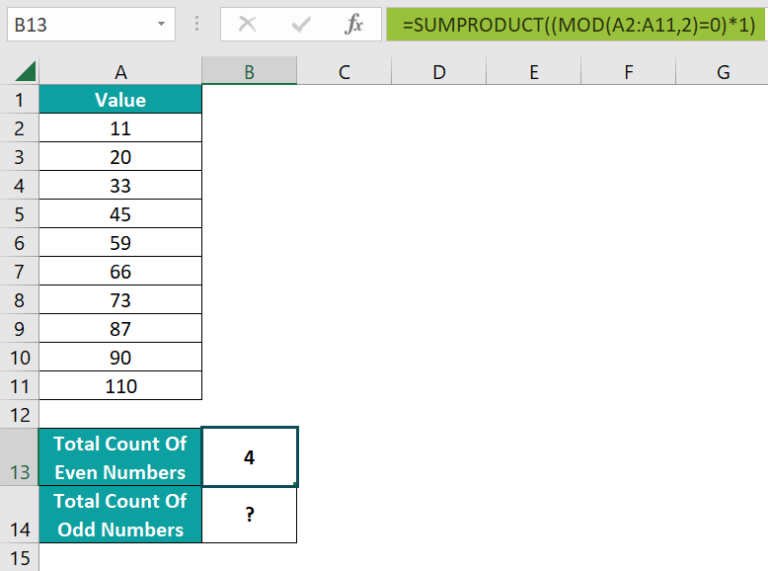• Step 2: Select the target cell B14, enter the below formula, and press Enter.
=SUMPRODUCT((MOD(A2:A11,2)=1)*1)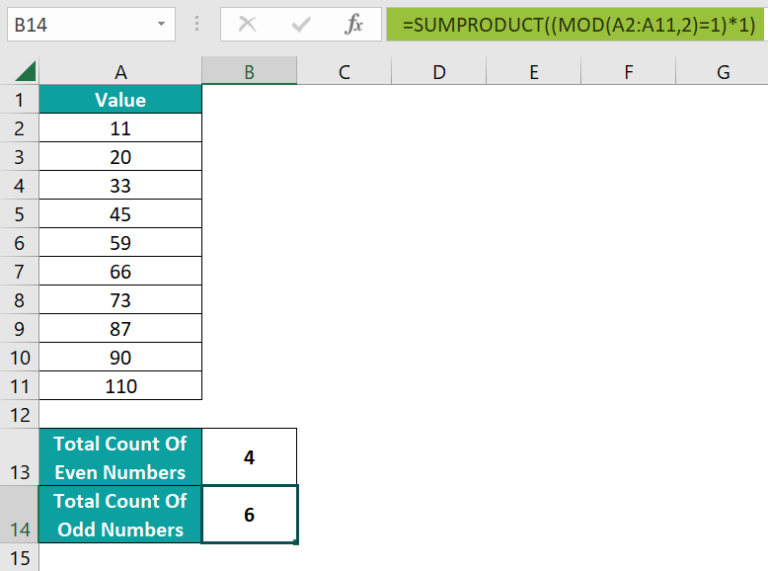The MOD() in the above formulas returns the remainder values obtained from dividing each number in the list by 2.

Then, the cell B13 formula checks whether the MOD() output is 0 to confirm if the specific column A cell value is even. The result will be an array of TRUEs and FALSEs, where TRUE indicates the particular cell value is even.

And multiplying the array range with the value of 1 will return an array of ones and zeros. Finally, the SUMPRODUCT() adds the array values to return the total count of even numbers, 4.

The cell B14 formula also works similarly, except the formula checks if the MOD() output is 1 to confirm the specific column A cell value is odd.

4. How can you represent the MOD() in terms of the INT()?

We can represent the MOD() in terms of the INT() as shown below:

MOD(number,divisor) = number – divisor * INT(number/divisor)